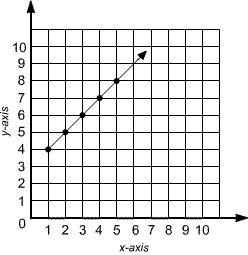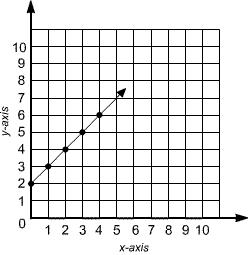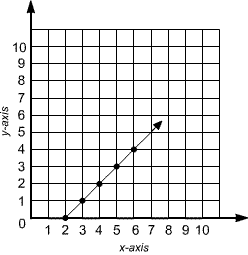Skill 10D Coordinate Graph   1. Use the following equation to complete the table. y = 2x + 2 x 0 1 2 3 4 y 2 4 6 2. Choose the graph that shows the equation y = x + 2 A. B.C. D.